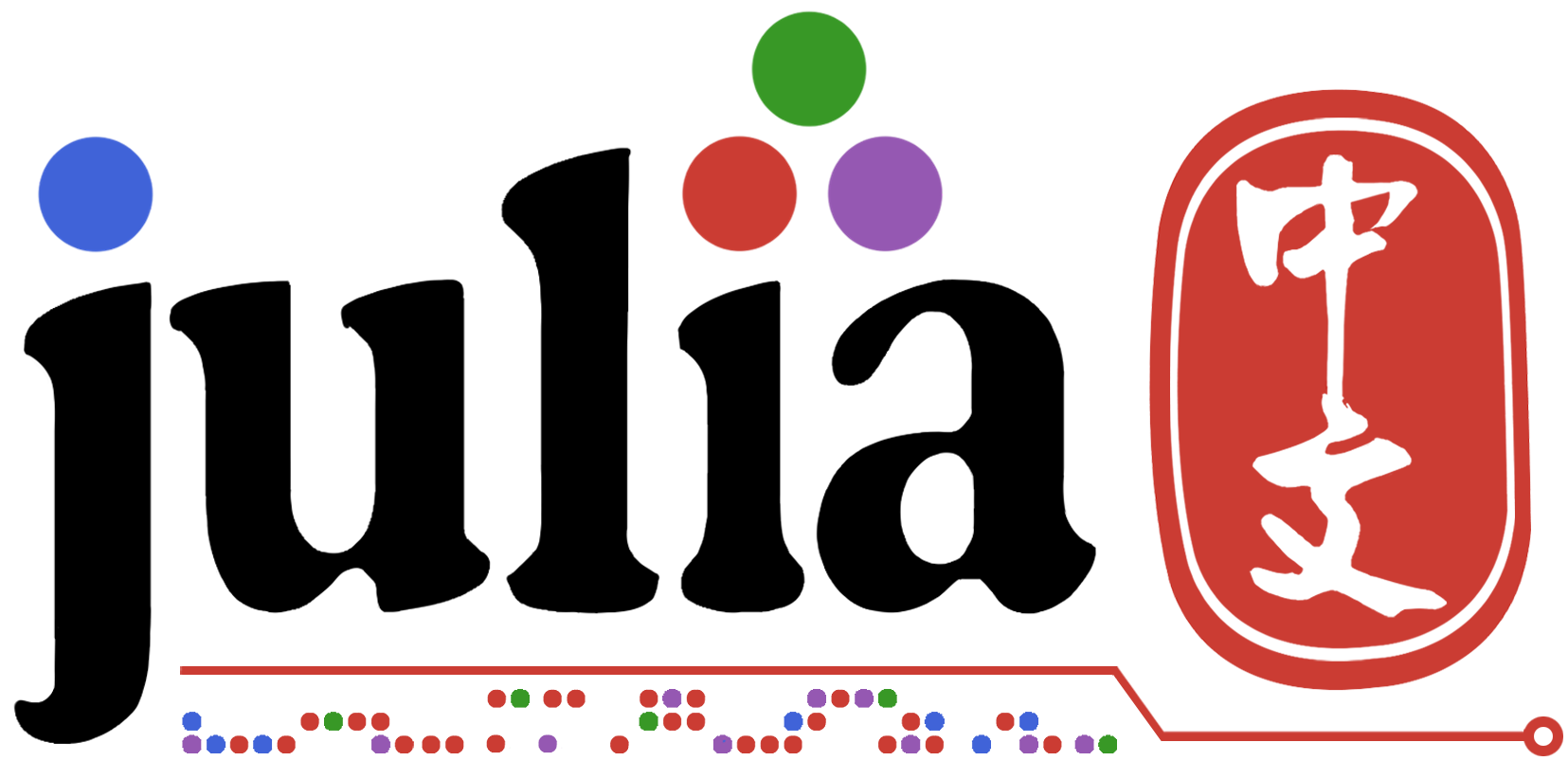# 概要

• Julia 有没有添加这些特性的考虑
• 或者说怎么向 Julia 建议这类想法
• 此外，这类特性是否会带来什么损失？
• 放在 Base 中的函数有什么要求，多了会带来什么影响？

• 给支持函数式编程的函数添加特性
• 添加新函数

# 添加特性

Julia 中的函数是“一等公民”，也即函数与普通变量平等看待。但有一些函数式编程特性 Julia 没有使用，或者说没有完全使用。

## 逻辑运算

1. Julia 的 `!, >, in` 等逻辑运算符支持函数式，比如
``````f = i -> true
!f # 等同于 (args...) -> !(f(args...))
>=(2) # 等同于 i -> (i >= 2)
``````
可以考虑新增的运算 `&&, ||`
``````f && g # 等同于 i -> f(i) && g(i)
## 使用场景
filter(>=(3) && <=(6), 1:100) # 返回 [3, 4, 5, 6]
findall(in([1,2,3]) || <(0), data)
``````

## 柯里化

``````filter(>(3), 1:10)
``````

``````f = filter(>(3))
f(1:10)
f(2:9)
``````

``````filter(f::Function) = data - > filter(f, data)
``````

p.s. 这些只是简单的修改，但可以更好地发挥函数式的编程特性

# 添加函数

1. NestWhile函数，迭代直到判断为否

``````function nest_while(f::Function, val::T, chk::Function)::T where T
while chk(val)
val = f(val)
end
val
end
``````
2. NestWhileList 函数，将过程结果一起返回

``````function nest_while_list(f::Function, val::T, chk::Function)::Vector{T} where T
res = [val]
while chk(val)
val = f(val)
push!(res, val)
end
res
end
``````
• 例1：`nest_while_list(i->i//2, 100, >=(1))` 返回值为 `[100, 50, 25, 12, 6, 3, 1, 0]`
• 例2：一些 LeetCode 题，比如 172，代码可以简化到一行
``````trailing_zeroes(n::Int) = sum(nest_while_list(i->i÷5, n÷5, >(1)))
``````
3. Nest 函数，比如 `mma_nest(f, val, 3) => f(f(f(val)))`

``````function mma_nest(f::Function, val::T, times::Int)::T where T
## e.g. mma_nest(f, val, 3) | returns f(f(f(val)))
for _ in 1:times
val = f(val)
end
val
end
``````
4. NestList 函数，将过程结果一起返回

`````` function mma_nest_list(f::Function, val::T, times::Int)::Vector{T} where T
## e.g. mma_nest(f, val, 2) | returns [val, f(val), f(f(val))]
res = Vector{T}(undef, times + 1)
res = val
for i in 2:(times + 1)
res[i] = val = f(val)
end
res
end
``````

比如 `mma_nest_list(f, val, 2) => [val, f(val), f(f(val))]`

``````# nest
reduce(1:times; init=val) do x, _
x = f(x)
end

# nest_list
reduce(1:times; init=[val]) do x, _
push!(x, f(x[end]))
end
``````

`NestWhile` 的话因为将 reduce 和 终止条件混合起来了，似乎没有现成的函数可以覆盖这个功能，我觉得可以试着在上游开一个 issue 提一下

3 个赞

2 个赞

Currying 的包有 GitHub - Orbots/Curry.jl: Currying for Julia ，不过没有注册。但我觉得 Julia 比较难以做 Currying 的原因是你如何和 optional arguments 的函数作区分呢？还有如果一个函数可以接受2个参数，也可以接受3个参数，那么你给2个参数的时候，Julia 怎么知道你想返回的是那个2个参数的函数的结果，还是一个 Currying 的函数呢？

• `filter` 用于筛选，而指定参数的 `filter(>(2))` 用于“筛选大于 2 的数”
• `in` 只是空泛的包含判断，`in(data)` 用于 `data` 的元素判断

``````f = Base.Fix2(-, 1)
f(3) # 3 - 1
f() # -1
``````

1 个赞